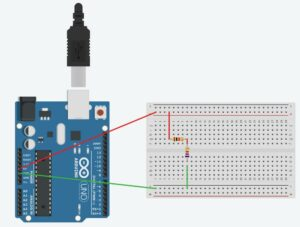## How to solve resistors in parallel and series?

We start furthest from the power inputs.  The orange box has the first two.  They are in series.  So we add them

R34 = R+ R4

R34 = 1kΩ + 1kΩ = 2kΩ

Now we move on to the next two

The next orange box has the two resistors in parallel

1/R234 = 1/R+ 1/R34

1/R234 = 1/1kΩ + 1/2kΩ

1/R234 = 1 + 1/2 = 3/2

R234= 0.67kΩThe last two resistors are in series

RTotal = R+ R234

RTotal = 1kΩ + 0.67kΩ = 1.67kΩ